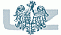1st WORKSHOP in MATHEMATICS, Lubiatów 1993
GRAPH THEORY Section

H. Bielak
Chromatically equivalent/unique graphs
M. Borowiecki and P. Mihók
Structure of hereditary properties of graphs
W. Chojnacki
On the chromatic number of some generalizations of permutation graphs
M. Horňák
A property of multi-p gonal and multi-q valency decompositions of the sphere
L.T.Q. Hung
The bandwith problem of caterpillars and tolerance graphs
S. Jendroľ
From combinatorial properties of convex polyhedron
M. Kwaśnik
On (3,1)-kernels in trees
D. Michalak
A characterization of perfect domination graphs
A. Rycerz
M-dimensional Frobenius problem and its applications
G. Stachowiak
Minimal change generalization algorithms and related enumeration problems
H.-J. Voss
Many short cycles in graphs with girth at least 5
A. Włoch
Sufficient conditions for a digraph to be (k,k-1)-kernel-perfect
I. Włoch
On minimal digraphs having (k,l)-kernels

Faculty of Mathematics, Informatics and EconometricsUniversity of Zielona Góra